# How to Sum by SUMPRDUCT with One Specific Criteria Multiple Columns in Excel

Sometimes we may meet the case that to sum numbers based on one specific criteria. In this article, we will show you the method to resolve this problem by formula with the help of Excel SUMPRODUCT function. SUMPRODUCT can filter data based on the supplied specific criteria and then sum up the filtered numbers properly.

Through a simple instance below, we will introduce you the syntax, argument and the usage of SUMPRODUCT function. We will let you know how the formula works step by step to reach your goal clearly. After reading the article, you may have a simple understanding of SUMPRODUCT function.

## EXAMPLE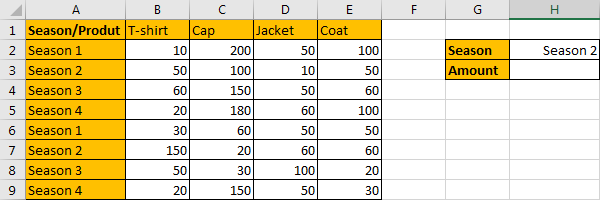Refer to the left-hand table, we can see it is a 2D table. It contains “Product” and “Season” two criteria, and range B2:E9 shows the amount for different products in different seasons. In the right-hand table, it is a simple summary table, it displays the total amount based on different season. As we can see in this case, we only want to sum total amount for season 2, so, there is only one condition “Season=Season 2”, we need to sum data for season 2 (in row3 and row7 but all columns).

Actually, user can also change season parameter in H2, it is a dynamic value. When applying the formula, we just use cell reference H2 to represent the value, if H2 value is changed, the returned value is also changed accordingly.

As we want to sum numbers from range B2:E9 based on the criteria “Season=Season 2”, we need to find out all numbers that can meet our requirement, and then sum up. To resolve this issue by formula, we can apply Excel SUMPRODUCT function.

## FORMULA – APPLY SUMPRODUCT FUNCTION

Step 1: Select A2:A9, then in Name Box define a name for this column, just use the column header ‘Season’.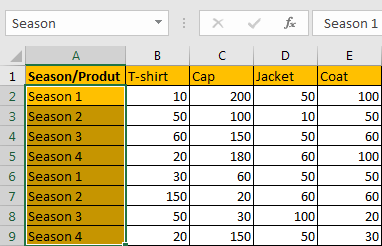Step 2: Select B2:E9, in Name Box define a name for this range, for example ‘Data’.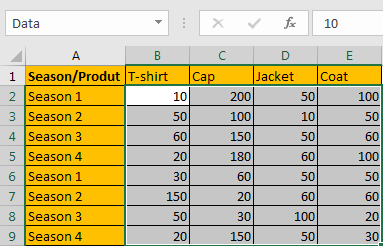Note: Above two steps are optional, you can directly use cell or range reference like A2:A9, B1:E1 in your formula, but for understanding well, we name ranges in advance.

Step 3: In H3, enter the formula =SUMPRODUCT(Data*(Season=H2)).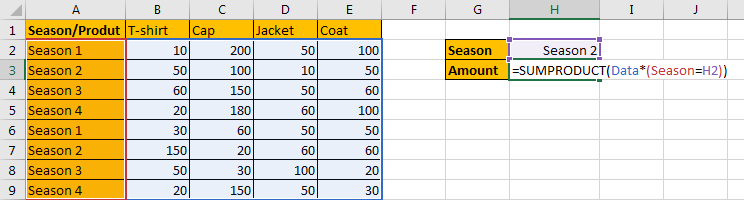Note: In above steps we already name ranges “Season” and “Data”, so when entering the range information into the formula, just after typing “Da…”, our named range “Data” is loaded, just select it from dropdown list.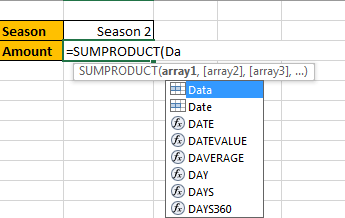Step 4: Press Enter after typing the formula.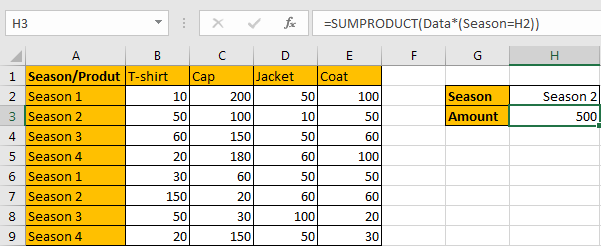We can see that data in row 3 and row 7 (multiple products columns) can meet our condition (Season=Season 2), so, values from all columns in row 3 and row 7 are summed, the total amount is 50+100+10+50+150+20+60+60=500. The formula works correctly.

## SUMPRODUCT FUNCTION INTRODUCTION

SUMPRODUCT function can be seen as SUM+PRODUCT.

For SUMPRODUCT function, the syntax is:

=SUMPRODUCT(array1,array2,array3, …)

The values in arrays are multiplied correspondingly, then sum up the products. For example, enter =SUMPRODUCT({1,2,3},{2,3,4}) in any cell, the we can get value 20, it equals to 1*2+2*3+3*4=20.

SUMPRODUCT function allows entering texts, logical operators and expressions like (product=cap), texts should be enclosed into ().

### SUMPRODUCT ARGUMENTS EXPLAINATION

SUMPRODUCT – ARRAY 1 – SUM RANGE

Refer to the left table, we know that the main range is “Data”, it records the amounts for each product in different seasons. In this range, we filter data by (Season=Season 2), then sum up the filtered data.

In the formula bar, select “Data”, press F9, we can see that values from range B2:E9 are listed properly. Each row is a group, and different groups are separated by “;” in the array.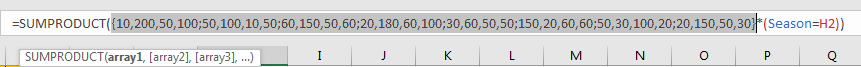SUMPRODUCT – ARRAY 1 – CRITERIA

In the right table, we know that there is only one condition that (Season=Season 2), so we enter (Season=H2). We can also change to another season in H2, the returned value will be updated based on the changing.

In the formula bar, select “Season”, press F9, and then select “H2”, press F9. All seasons are listed in an array. Then we get a logical expression ({“Season 1″;”Season 2″;”Season 3″;”Season 4″;”Season 1″;”Season 2″;”Season 3″;”Season 4″}=”Season 2”).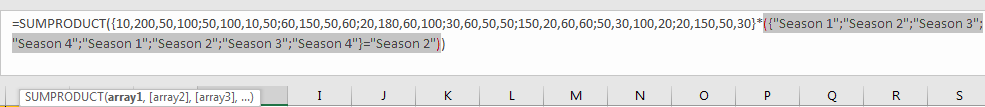### SUMPRODUCT WORKFLOW

After explaining argument in the formula, now we will show you how the formula works with the help of these arrays and expressions.

`=SUMPRODUCT({10,200,50,100;50,100,10,50;60,150,50,60;20,180,60,100;30,60,50,50;150,20,60,60;50,30,100,20;20,150,50,30}*({"Season 1";"Season 2";"Season 3";"Season 4";"Season 1";"Season 2";"Season 3";"Season 4"}="Season 2"))`

Select the logical expression in the formula bar, press F9. We can get result array combined with “True” and “False”:

`=SUMPRODUCT({10,200,50,100;50,100,10,50;60,150,50,60;20,180,60,100;30,60,50,50;150,20,60,60;50,30,100,20;20,150,50,30}*{FALSE;TRUE;FALSE;FALSE;FALSE;TRUE;FALSE;FALSE})`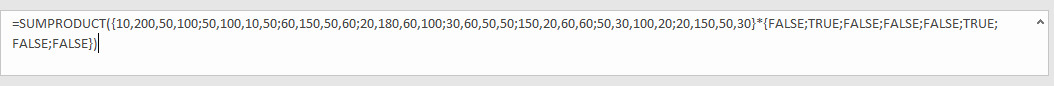Select the two arrays, and press F9:

`=SUMPRODUCT({0,0,0,0;50,100,10,50;0,0,0,0;0,0,0,0;0,0,0,0;150,20,60,60;0,0,0,0;0,0,0,0})`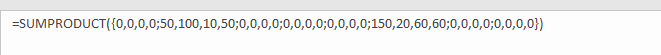Then sum up all the values in this array. We get 50+100+10+50+150+20+60+60=500 at last.

### NOTE

In workflow, for criteria {FALSE;TRUE;FALSE;FALSE;FALSE;TRUE;FALSE;FALSE}, we can convert ‘True’ to ‘1’ and ‘False’ to ‘0’, then we get an array {0,1,0,0,0,1,0,0} which consists of numbers.

Then we have below formula:

`=SUMPRODUCT({10,200,50,100;50,100,10,50;60,150,50,60;20,180,60,100;30,60,50,50;150,20,60,60;50,30,100,20;20,150,50,30}*{0,1,0,0,0,1,0,0})`

It can be seen as values in each row multiplied by the values in array {0,1,0,0,0,1,0,0} accordingly.

{10,200,50,100} * {0}

{50,100,10,50} * {1}

{60,150,50,60} * {0}

{20,180,60,100} * {0}

{30,60,50,50} * {0}

{150,20,60,60} * {1}

{50,30,100,20} * {0}

{20,150,50,30} * {0}

=>

{0,0,0,0}

{50,100,10,50}

{0,0,0,0}

{0,0,0,0}

{0,0,0,0}

{150,20,60,60}

{0,0,0,0}

{0,0,0,0}

=>

{0,0,0,0;50,100,10,50;0,0,0,0;0,0,0,0;0,0,0,0;150,20,60,60;0,0,0,0;0,0,0,0}

### SUMMARY

1.  SUMPRODUCT function can handle multiple arrays, and it sum up the products of these arrays.

2. SUMPRODUCT function allows user defined range, wildcards, logical operators, expressions.

### Related Functions

• Excel SUMPRODUCT function
The Excel SUMPRODUCT function multiplies corresponding components in the given one or more arrays or ranges, and returns the sum of those products.The syntax of the SUMPRODUCT function is as below:= SUMPRODUCT (array1,[array2],…)…